AVL Racing Models Shock Absorbers for Race Cars

Today’s guest bloggers are Marco Scheiber and Eduardo Gimenez from AVL Racing. They are here to talk about the modeling of shock absorbers for race cars.

Introduction

Recently, a master’s thesis regarding damper modeling and the approach to consider the non-linearity and transient behavior of hydraulic race car dampers was executed in the racing department of the AVL List GmbH. Dampers or shock absorbers are known to be mainly velocity depended devices, but they are also dependent on oil compressibility and other parameters which typically lead to hysteretic damping characteristics. As physical damper models are typically suitable only for one specific damper architecture, we focused on empirical/mathematical models based on measurement data logged by damper dynamometers. From a variety of investigated damper modeling approaches, one approach, basically known as the restoring force method , is presented in the next few pages.

Motivation

Accurate vehicle models are needed to achieve a good correlation between simulation and reality. Furthermore, the main motivation in a lap time simulation is to give us an opportunity to predict how the vehicle will perform on a certain racetrack for a given setup. The purpose of dampers is to reduce the oscillations of the chassis and wheels respectively. As the contact patch and therefore friction coefficient between tires and the track surface is highly influenced by the vertical wheel load variations, dampers are designed to reduce the load variations to a minimum and to have adequate body control, mainly in high downforce race cars. Accurate damper models are needed, to better understand the effects of the damping forces on vehicle dynamics. Typically, conventional mathematical damper models do not consider hysteretic effects on the damping force, mainly coming from the oil’s compressibility. Therefore, we decided to look for opportunities to take the hysteresis of shock absorbers into account within mathematical models. Figure 1 illustrates the purpose of a lap time simulation and the main requirements of our damper model.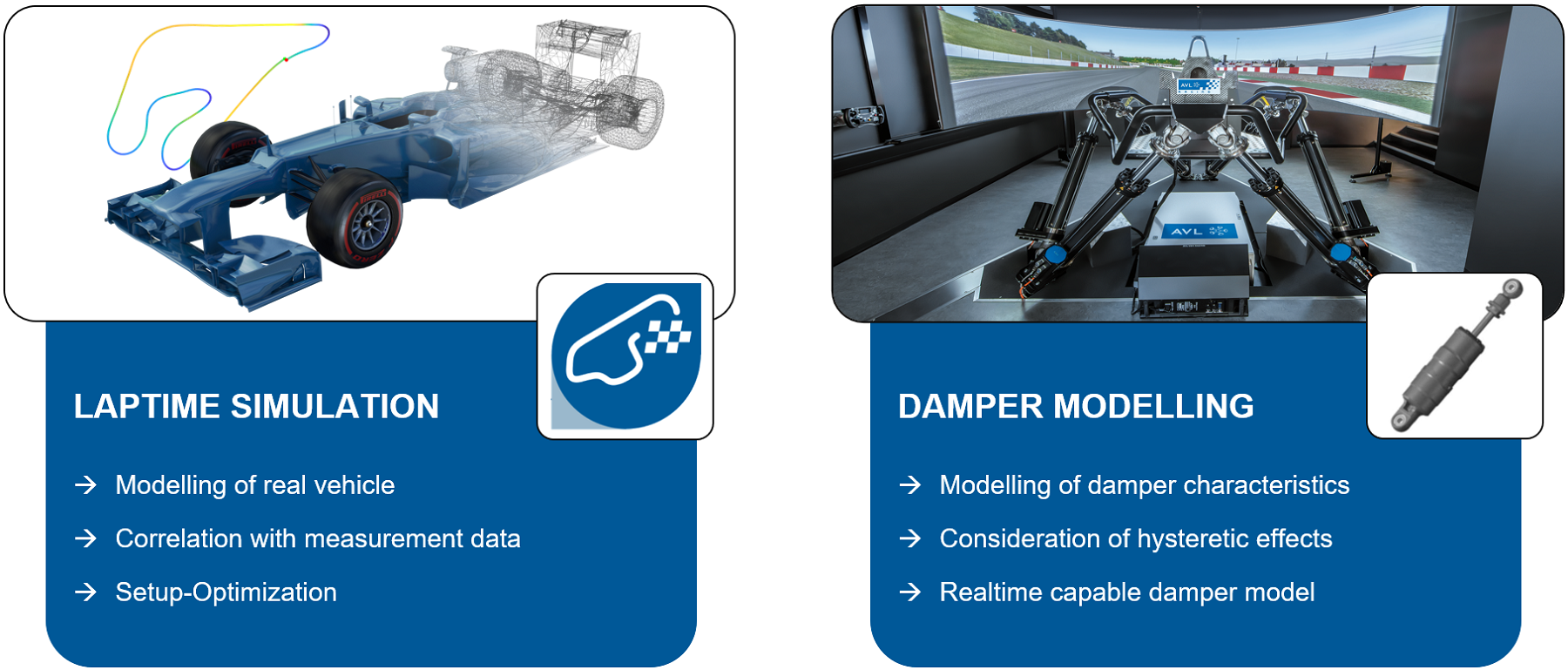Figure 1: Damper Modelling – Motivation

Methodology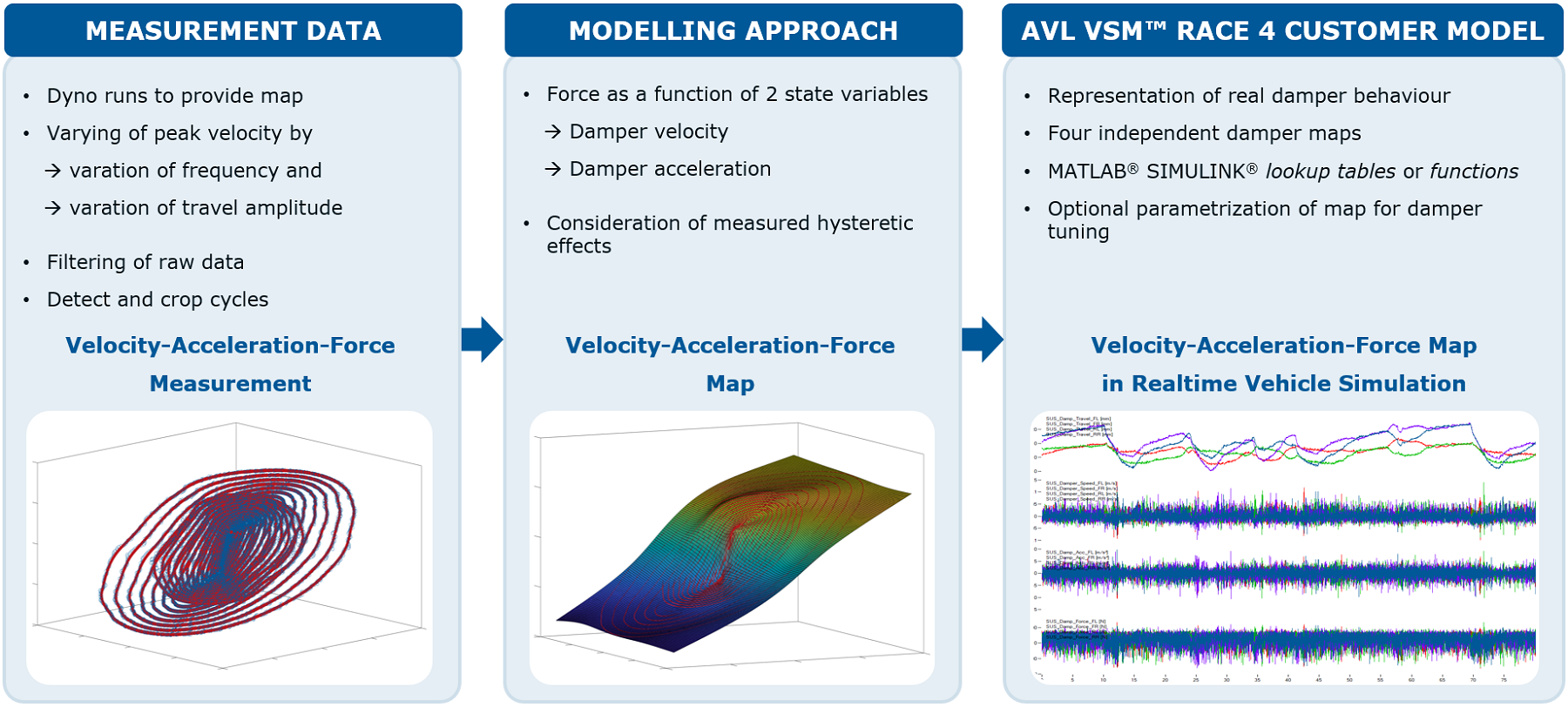Figure 2: Force State Map Approach – Model Overview

Based on the observations of Duym , we used the restoring force method for modeling purposes. Figure 2 depicts a general overview and usage of a restoring force model in a vehicle simulation. To apply the restoring force method and the force state map respectively, several sinusoidal damper dynamometers run need to be executed. The objective of the measurement series is to provide a wide range of peak velocities and peak accelerations by varying the excitation frequency and travel amplitude. Further processing of the measurement data includes the usage of signal filters. Additionally, each cyclical run is divided and cropped into cycles and only one cycle is used for the model generation. To consider hysteretic effects measured by shock testers, the force is described as a function of two state variables, the damper velocity and the damper acceleration. Finally, we implemented the generated models in our transient vehicle simulation AVL VSM™ RACE 4.

As the model accuracy prior depends on the surface fitting algorithm, we executed and compared several approaches to solve the fitting problem. Figure 3 illustrates two exemplary surface fitting methods which are applied. The models are labeled as CL Curve Fit and ML Curve Fit respectively. The model denotation is attributed to internal nomenclature for two different types of fitting algorithms. We used the gridfit function  for our first model while for the second model, the MATLAB® curve fitting toolbox  was used.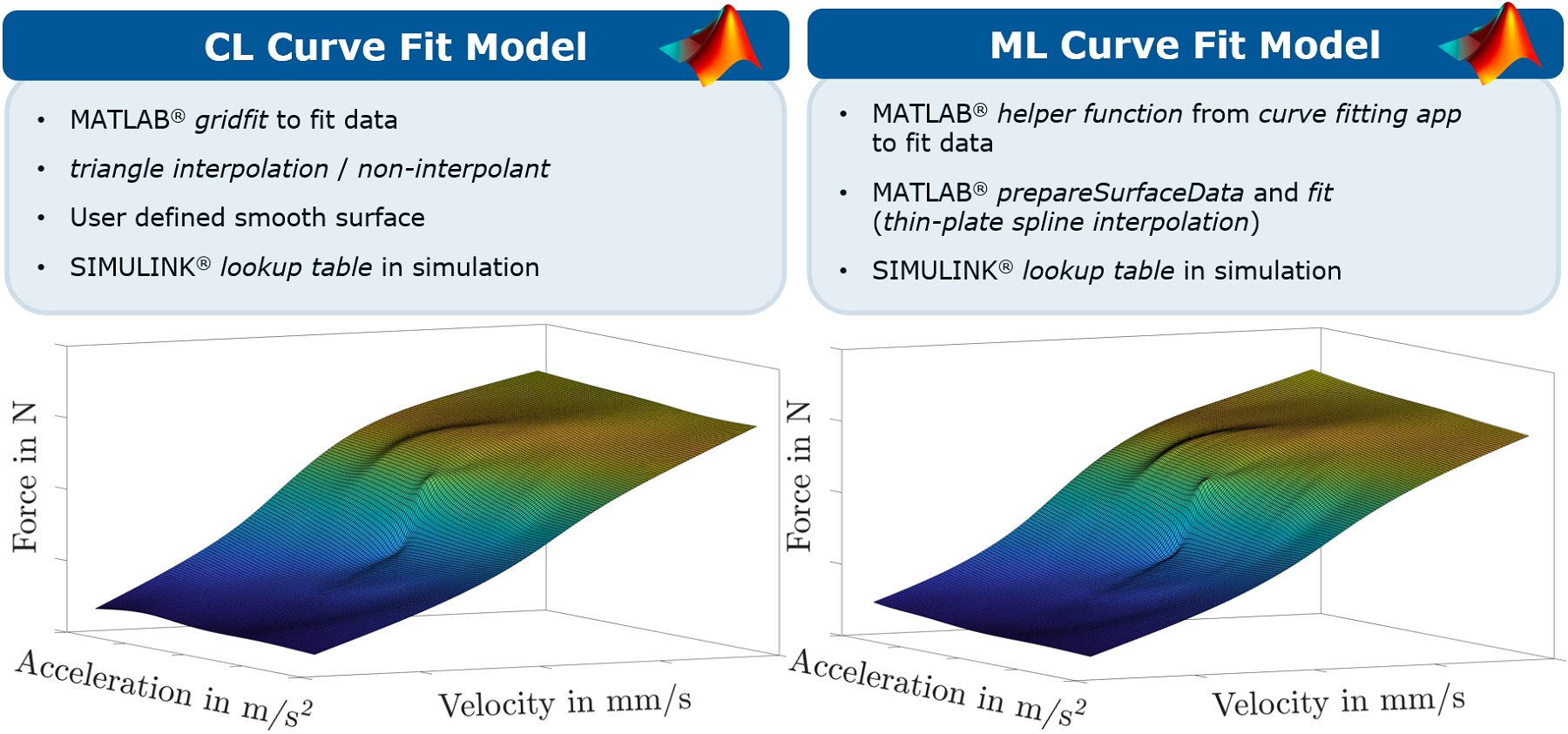Figure 3: Used MATLAB® Fitting Algorithms

Model Validation

We calculated root mean square errors to validate the models and to get a first general indication on how good the surfaces fit to the measured data. Additionally, we determined the fitting times which are also depicted in Figure 4. While the RMSE of both force state maps are almost identical, the fitting time of the CL Curve Fit Model is considerably faster than the fitting time of the ML Curve Fit Model.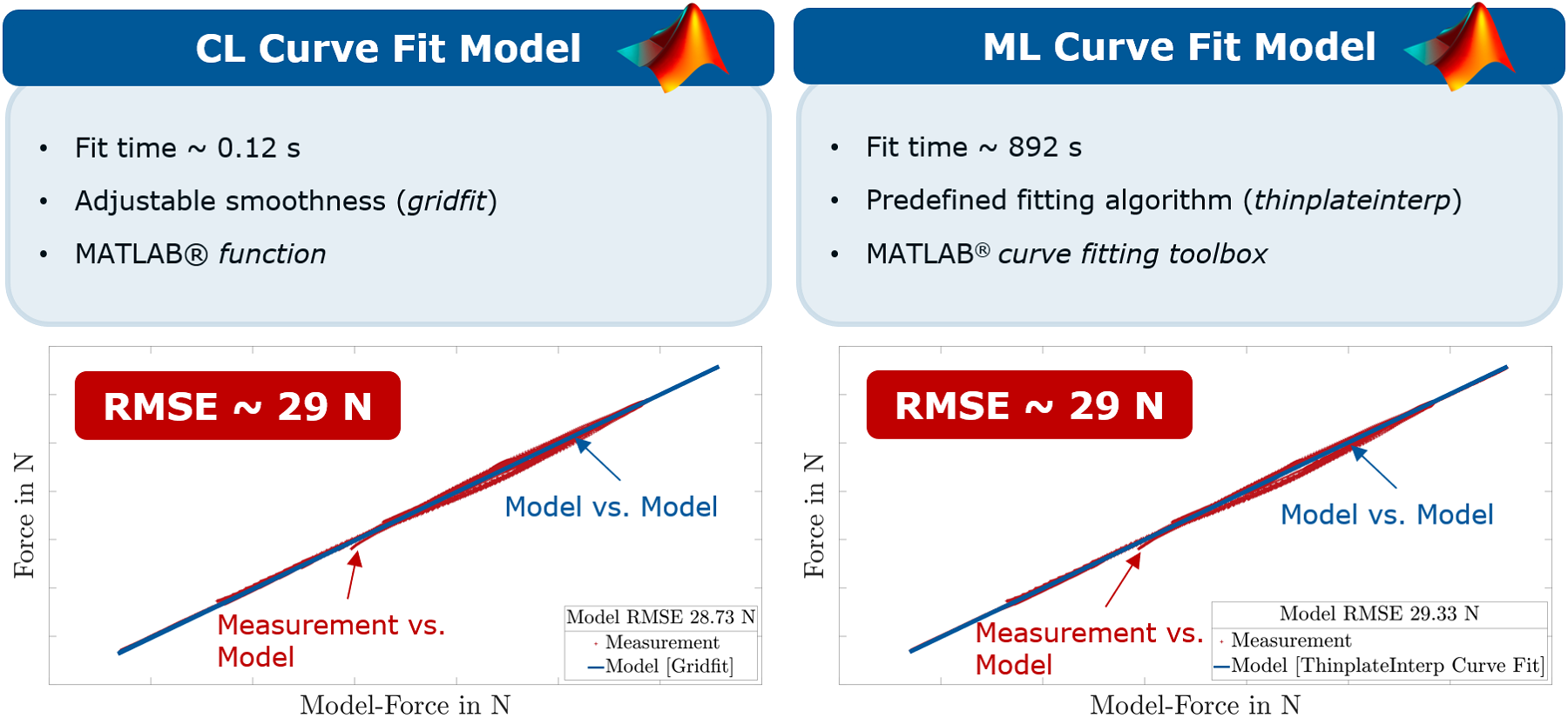Figure 4: Force State Map Models – Map Validation

The validation results of the entire measurement series of the first damper are visually summarized in Figure 5. The data has been sorted according to the chronological order of the measurements. On the x-axes of this illustration, the excitation frequency and therefore the peak velocity increases until the stroke changes. At this point, the procedure is repeated. Firstly, it can be noticed, that the RMSE of the CL Curve Fit model and the ML Curve Fit Model are below 51 N for the entire measurement series. Mostly the error is even smaller than that. In general, the CL Curve Fit Model tends to fit slightly better than the ML Curve Fit Model. The fit errors of all force state map models tend to increase at lower peak velocities referring to both depicted strokes.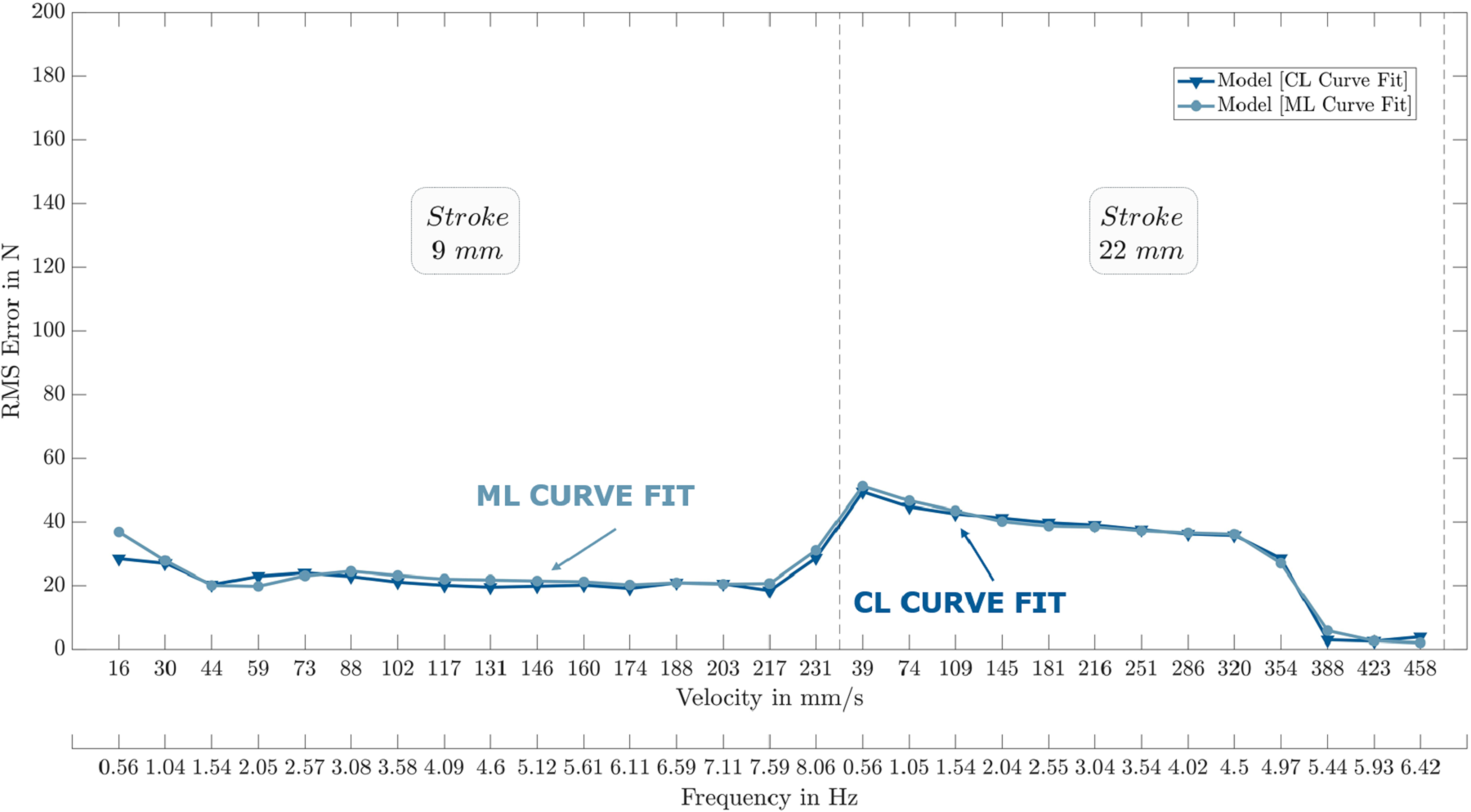Figure 5: Force State Map Models – Single Run Validation – damper 1

The overall validation of the models is illustrated for a second shock absorber in Figure 6. It can be noticed that the RMSE of the CL Curve Fit Model and the ML Curve Fit Model are below 21 N for the entire measurement series. In general, the ML Curve Fit Model does slightly better than the CL Curve Fit Model.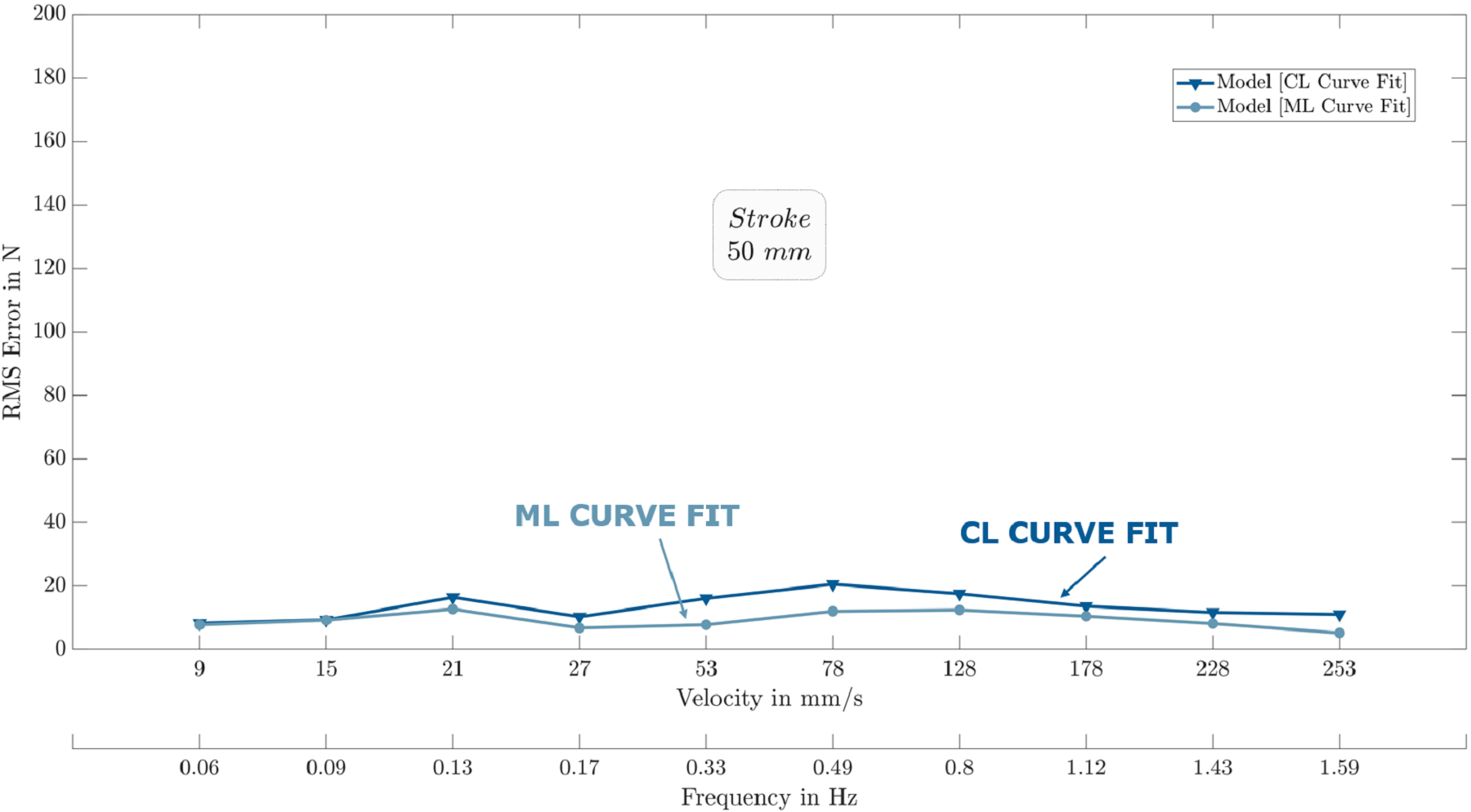Figure 6: Force State Map Models – Single Run Validation – damper 2

Model Implementation

The generated models are implemented in the transient vehicle simulation AVL VSM™ RACE 4 by using lookup tables and S-function blocks in a virtual MATLAB®/ Simulink® environment. Figure 7 depicts a general overview of the Vehicle Simulation Model (VSM) and the AVL Customer Model.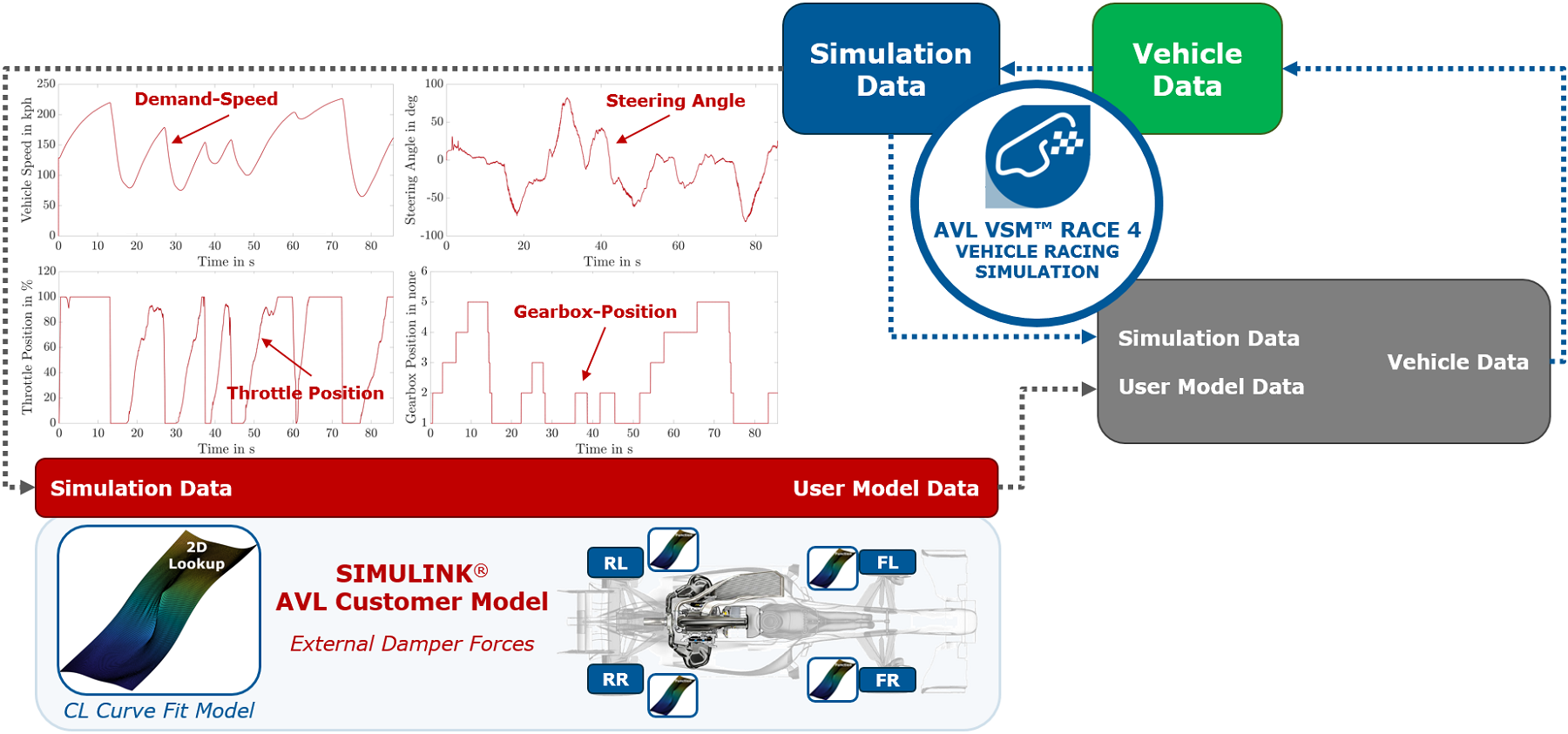Figure 7: Overview – Vehicle Simulation Model

Finally, the areas of operation in the three-dimensional force state maps of the simulated vehicle are shown in Figure 8.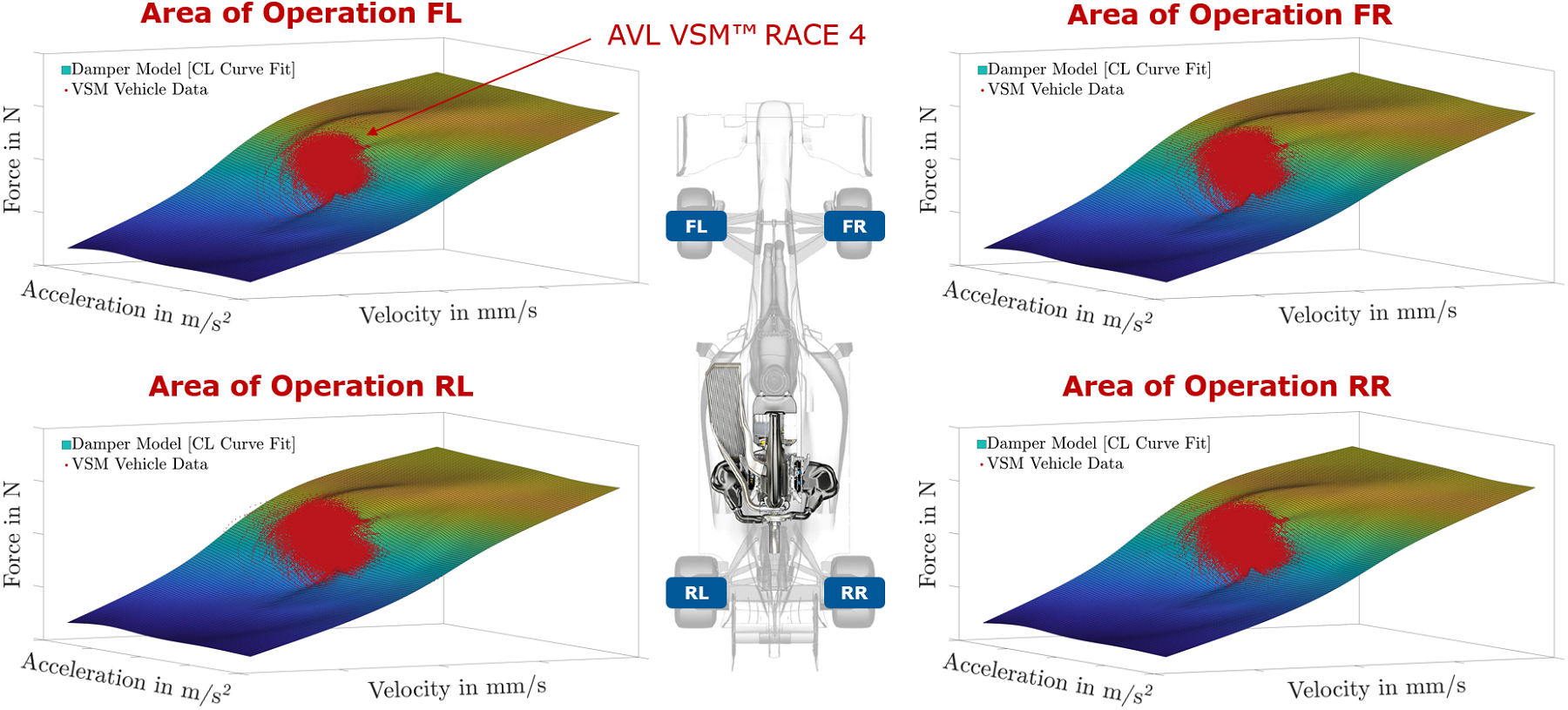Figure 8: Areas of Operation in Force State Maps – CL Curve Fit

Conclusion and Perspective

We found that the force state map approach can capture the damper’s non-linearity and was able to model the behavior of different shock absorber types with high accuracy. The damper maps allowed us to model individual shock absorbers without knowing their specific component geometries. As empirical models depend on field observations, damper dynamometers were necessary prior to the model generation. Furthermore, the acceleration dependent hysteretic behavior of dampers was considered by this approach which is neglected by simple force-velocity damper models.

By using the gridfit function the measurement data could be fitted much faster than the thin plate-spline interpolation method from the curve fitting toolbox. RMSE was reasonably low and similar in both investigated approaches. Also, we found that the thin plate-spline interpolation model tended to overfit the measurement, especially if a lower number of dynamometer runs were executed.

Parametrizing the force state maps give us the opportunity to run a variety of simulation runs in our AVL-Cloud Client application to find the theoretical optimum of user defined performance parameters. A map parametrization example can be found in Bedük et. al. . Typically, the optimum can be determined not only by objective parameters but also subjective feedback from professional racing drivers. The subjective evaluation can be done by using the models on our AVL RACING DRIVER in the LOOP (DiL) simulator. Besides performance investigations on racecars, the variation of damper map parameters can be also used to investigate the ride comfort in the passenger car application.

For each individual damper, further investigations on the effect of the oil temperature on the damping force are needed. Depending on the damper design, the temperature operating range can change to a various extent.

References

1. AVL List GmbH. AVL DRIVE RACE User Manual. AVL List GmbH, Graz, Austria, 2020. https:\\www.avl.com
2. M. Trzesniowski. Fahrwerk. Handbuch Rennwagentechnik. Springer Vieweg, Wiesbaden, 2019.
3. S. Duym. An alternative force state map for shock absorbers. Proceedings of the Institution of Mechanical Engineers, Part D: Journal of Automobile Engineering, 211(3):175-179, 1997.
4. J. D’Errico. Surface Fitting using gridfit (https://www.mathworks.com/matlabcentral/fileexchange/8998-surface-fitting-using-gridfit), MATLAB Central File Exchange. Retrieved August 12, 2021.
5. The MathWorks, Inc. Curve Fitting Toolbox, 2021. Available at https://www.mathworks.com/help/curvefit/index.html?s_tid=CRUX_lftnav, Accessed on 14 March 2021.
6. D. Madier. The Formula 1 Hybrid Power Units 2014-2015: F1 Season 2015, 2016. Available at https://www.f1-forecast.com/pdf/The%20Formula%201%20Hybrid%20Power%20Units%202014-2015.pdf, Accessed on 04 April 2021.
7. M. D. Bedük, K. Çalışkan, R. Henze, and F. Küçükay. Advanced parameter analysis for damper influence on ride dynamics. Journal of Vibration and Control, 24(8):1393-1411, 2018.
|